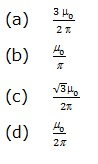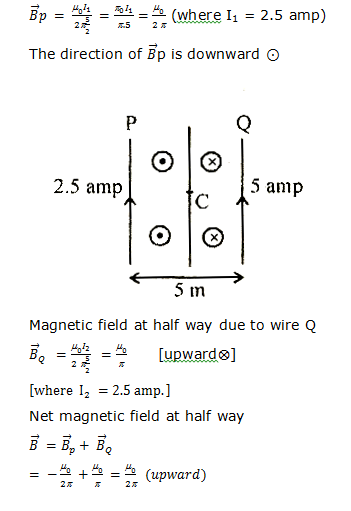# Two long parallel wires P and Q are both perpendicular to the plane of the paper with distance of 5 m between them. If P and Q carry currents of 2.5 amp and 5 amp respectively in the same direction, then the magnetic field at a point half-way between the wires is10 years ago

(d)

When the current flows in both wires in the same direction then magnetic field at half way due to the wire P,